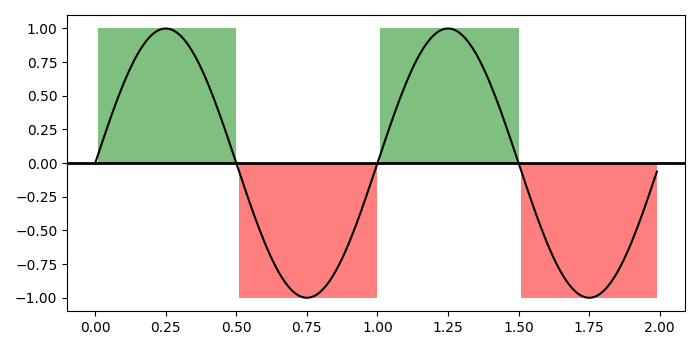# Make logically shading region for a curve in matplotlib

MatplotlibPythonData Visualization

To make logically shading region for a curve in matplotlib, we can take the following steps

## Steps

• Set the figure size and adjust the padding between and around the subplots.

• Create t, s1 and s2 data points using numpy.

• Create a figure and a set of subplots.

• Plot t and s1 data points; add a horizontal line across the axis.

• Create a collection of horizontal bars spanning *yrange* with a sequence of xranges.

• Add a '~.Collection' to the axes' collections; return the collection.

• To display the figure, use Show() method.

## Example

import numpy as np
import matplotlib.pyplot as plt
import matplotlib.collections as collections

plt.rcParams["figure.figsize"] = [7.00, 3.50]
plt.rcParams["figure.autolayout"] = True

t = np.arange(0.0, 2, 0.01)
s1 = np.sin(2 * np.pi * t)
s2 = 1.2 * np.sin(4 * np.pi * t)

fig, ax = plt.subplots()

ax.plot(t, s1, color='black')
ax.axhline(0, color='black', lw=2)

collection = collections.BrokenBarHCollection.span_where(t, ymin=0, ymax=1,
where=s1 > 0, facecolor='green', alpha=0.5
)

collection = collections.BrokenBarHCollection.span_where( t, ymin=-1, ymax=0,
where=s1 < 0, facecolor='red', alpha=0.5
)
plt.show()